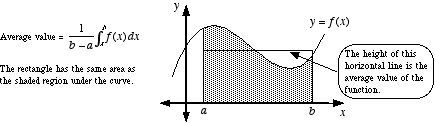# average value of a function

Average Value of a Function

The average height of the graph of a function. For y = f(x) over the domain [a, b], the formula for average value is given below.